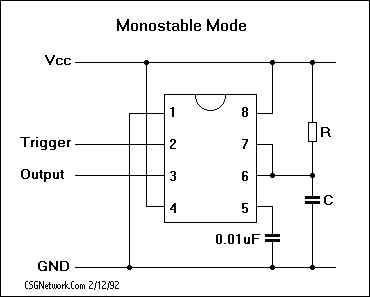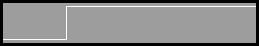# Monostable 555 Timeout Calculator

This calculator requires the use of Javascript enabled and capable browsers. This calculator is designed to give time out value (delay) for the 555 timer timer, based on the control capacitance and resistance. This particular configuration is for an monostable time delay calculation. When power is applied the output is low until the delay time has elapsed, the output then goes high and remains high. The formula is:

Time Out Delay (secs) = 1.1 * R * C

Where R is in Ohms and C is in F. Enter the capacitance of C in farads (not microfarads) and the resistance of R in Ohms. Click on Calculate to return the timeout in seconds. You might also wish to see our Astable 555 Square Wave Calculator.555 Calculator Required Data Entry Capacitor C Farads Resistor R Ohms Calculated Actual Results Delay Time Out Seconds Calculated Rounded Results Delay Time Out Seconds
Version 1.1.9

 Leave us a question or comment on FacebookSearch or Browse Our Site